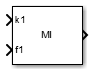# Matrix Interpolation

Interpolate between matrices

•Libraries:

## Description

The Matrix Interpolation block performs interpolation (or extrapolation) on an N-dimensional table by using pre-calculated indices and fraction values. Each data point can be a matrix. It supports interpolation up to three breakpoint dimensions. If you specify n dimensions for the breakpoints, and each data point in your table is a matrix that has m dimensions, then the number of dimensions for your table data is m+n. The last n dimensions of the table data must be the length of breakpoints in each interpolation dimension. The first m dimensions of the matrix are each a data point.

## Ports

### Input

expand all

Index `k1` for the first dimension of the table.

Data Types: `single` | `double`

Fraction `f1` for the first dimension of the table.

Data Types: `single` | `double`

Index `kn` for the n-th dimension of the table.

Data Types: `single` | `double`

Fraction `fn` for the n-th dimension of the table.

Data Types: `single` | `double`

### Output

expand all

Approximation of N-dimensional function, computed by interpolating (or extrapolating) table data using values from the input index, `k`, and the fraction, `f`.

Data Types: `single` | `double`

## Parameters

expand all

Choose how to interpolate table data in between breakpoints. Select `Flat`, `Nearest`, or `Linear`. For more information, see Interpolation Methods.

#### Programmatic Use

 Block Parameter: `InterpMethod` Type: character vector Values: `'Flat' | 'Nearest' | 'Linear'` Default: `'Linear'`

Choose how to extrapolate table data if your index k and fraction f from the inputs are beyond the range of the breakpoints. Select `Clip` or `Linear`. For more information, see Extrapolation Methods.

#### Programmatic Use

 Block Parameter: `ExtrapMethod` Type: character vector Values: `'Clip' | 'Linear'` Default: `'Clip'`

Enter the number of dimensions of the lookup table by specifying an integer from `1` to `3`. This parameter determines:

• The number of independent variables for the table and the number of block inputs.

• The number of breakpoint input sets to specify.

#### Programmatic Use

 Block Parameter: `InterpolateDimension` Type: character vector Values: `'1' | '2' | '3'` Default: `'1'`

Enter the table of output values. The number of dimensions of the table data must be the sum of the number of breakpoint dimensions and the number of matrix dimensions because each data point is a matrix for this block.

For information about how to construct multidimensional arrays in MATLAB®, see Multidimensional Arrays.

#### Programmatic Use

 Block Parameter: `TableData` Type: character vector Values: vector | matrix | N-D array Default: `'1:1:10'`

Select the simulation mode.

• `Code generation` — On the first model run, simulate, and generate code for the MATLAB System block with only MATLAB functions supported for code generation. If the structure of the block does not change, subsequent model runs do not regenerate the code.

If the simulation mode is ```Code generation```, system objects accept a maximum of 32 inputs.

• `Interpreted execution` — Simulate the model by using all supported MATLAB functions. Choosing this option can slow simulation performance.

#### Programmatic Use

 Block Parameter: `SimulateUsing` Type: character vector Values: ```'Code generation' | 'Interpreted execution'``` Default: `'Code generation'`

## Version History

Introduced in R2016a